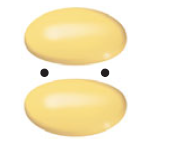×
Get Full Access to Chemistry: The Central Science - 12 Edition - Chapter 9 - Problem 85e
Get Full Access to Chemistry: The Central Science - 12 Edition - Chapter 9 - Problem 85e

×

# Molecular Orbitals and Period 2 Diatomic | Ch 9 - 85EISBN: 9780321696724 27

## Solution for problem 85E Chapter 9

Chemistry: The Central Science | 12th Edition

• Textbook Solutions
• 2901 Step-by-step solutions solved by professors and subject experts
• Get 24/7 help from StudySoup virtual teaching assistantsChemistry: The Central Science | 12th Edition

4 5 1 299 Reviews
18
1
Problem 85E

Consider the molecular orbitals of the P2 molecule. Assume that the MOs of diatomics from the third row of the periodic table are analogous to those from the second row. (a) Which valence atomic orbitals of P are used to construct the MOs of P2? (b) The figure that follows shows a sketch of one of the MOs for P2.What is the label for this MO? (c) For the P2 molecule, how many electrons occupy the MO in the figure? (d) Is P2 expected to be diamagnetic or paramagnetic? Explain.Step-by-Step Solution:
Step 1 of 3

Chem Study Guide Chapters 1 & 2 1.1 Chemistry- the study of matter and energy and the changes they undergo Matter- anything that has mass and occupies volume (space). 1.2 States of Matter solid- particles packed tightly together. Has a deﬁnite shape and a deﬁnite volume. liquid- particles are more spaced out. Has an indeﬁnite shape and a deﬁnite volume. gas- particles are spaced far apart. Has an indeﬁnite shape and an indeﬁnite volume. Physical Properties- can be observed or measured with your senses without changing the identity of the matter. ex) melting point (mp), boiling point (bp), solubility, color and odor. Chemical Properties- the ability to undergo chemical change.

Step 2 of 3

Step 3 of 3

##### ISBN: 9780321696724

This full solution covers the following key subjects: mos, Orbitals, figure, row, molecule. This expansive textbook survival guide covers 49 chapters, and 5471 solutions. The full step-by-step solution to problem: 85E from chapter: 9 was answered by , our top Chemistry solution expert on 04/03/17, 07:58AM. Since the solution to 85E from 9 chapter was answered, more than 449 students have viewed the full step-by-step answer. This textbook survival guide was created for the textbook: Chemistry: The Central Science, edition: 12. Chemistry: The Central Science was written by and is associated to the ISBN: 9780321696724. The answer to “?Consider the molecular orbitals of the P2 molecule. Assume that the MOs of diatomics from the third row of the periodic table are analogous to those from the second row. (a) Which valence atomic orbitals of P are used to construct the MOs of P2? (b) The figure that follows shows a sketch of one of the MOs for P2.What is the label for this MO? (c) For the P2 molecule, how many electrons occupy the MO in the figure? (d) Is P2 expected to be diamagnetic or paramagnetic? Explain.” is broken down into a number of easy to follow steps, and 90 words.

Unlock Textbook Solution